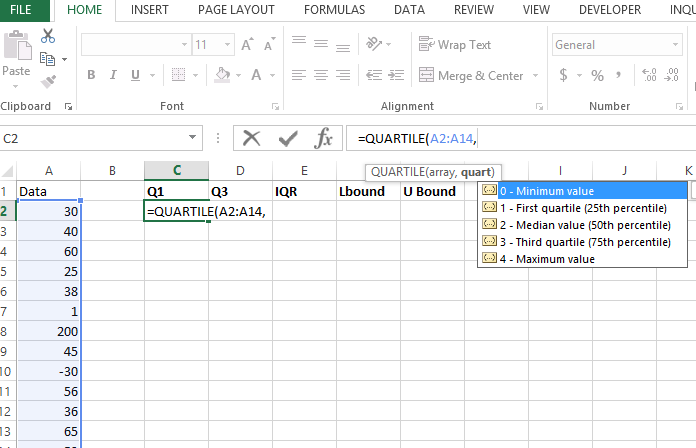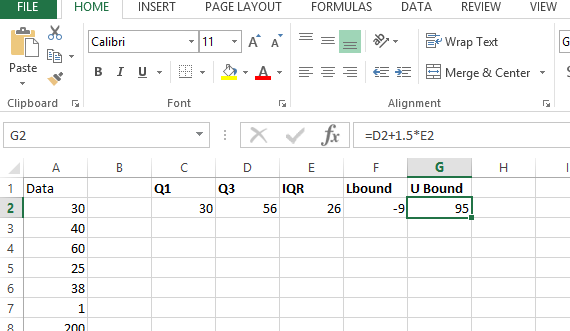## Finding Outliers in Excel

In this article we will learn how to find outliers in Excel.

What are Outliers?
Outliers are the values in a data which are outside the scope of the general data values, means which are very much higher than very much lower than the general data values.

Why do we need to remove the Outliers?
Outliers spoil our data and representation, when we create a graph of complete data if some values are extremely high and extremely low it other values / range of the graph does not look good.

Let us create simple data. In this example we can clearly see that some values are really outside e.g 200.Let discuss the procedure to remove outliers. Lets start by finding the first and 3rd quartile:

1st quartile: =QUARTILE(A2:A14,1)3rd quartile: =QUARTILE(A2:A14,3)Lets calculate interquartile range (IQR). Because it is a range you just need to subtract values: Q3-Q1.Calculating IQR gives us possibility to calculate lower and upper bound of data.

Lets us calculate the lower bound and upper bound values:

Lower bound = lower of Q1 or Q3 – 1.5 * IQRUpper bound = max of Q1 or Q3 + 1.5 * IQRLet us find the outlier by using the U bound and L bound: =OR(A2<\$F\$2,A2>\$G\$2)

Anything lower than lower bound and higher than upper bound is an outlier.

## Template

```Further reading: